Chinstrap Penguins, by Derek Oyen, Unsplash.

One of the key steps in the Exploratory Data Analysis process that comes before model development is to understand the statistical distribution of the variables or features within the data set. Visualising the statistical distribution of variables can tell you about the range that observations cover, what their central tendency is, whether they’re skewed in a particular direction, and whether there are outliers.

Seaborn, which is built on top of the Matplotlib library, makes it easy to take data from a Pandas dataframe and visualise the statistical distribution. You can use it for univariate, bivariate, and multivariate visualisation to help you gain a better understanding of the data. Here’s a quick guide to the types of visualisation you can use to examine statistical distributions.

For this project we’re going to use Pandas for displaying and manipulating text data and the Seaborn data visualisation package, which is built on top of Matplotlib. Open up a Jupyter notebook and import the packages.

``````import pandas as pd
import seaborn as sns
``````

Next, check which version of Seaborn you have installed. Some of the functions below are new additions to Seaborn, so you’ll need to install a recent version. I’m using version 0.11.0. You can upgrade Seaborn by entering `pip3 install --upgrade seaborn` into a code cell and then executing it using shift and enter.

``````sns.__version__
``````
``````'0.11.0'
``````

To make the charts look a bit crisper on 4K or retina displays, we’ll also configure a couple of Seaborn settings to increase the quality of the images generated, and we’ll define the figure size to avoid code repetition.

``````%config InlineBackend.figure_format = 'retina'
sns.set_context('notebook')
sns.set(rc={'figure.figsize':(15, 6)})
``````

We will use one of the built-in Seaborn datasets to save the hassle of finding and downloading one. The Penguins data set is nice and small and easy to understand, so we’ll load that into a Pandas dataframe and view the output. This data set contains taxonomic data on the meristic features of three different species of penguin.

``````df = sns.load_dataset('penguins')
``````
species island bill_length_mm bill_depth_mm flipper_length_mm body_mass_g sex
0 Adelie Torgersen 39.1 18.7 181.0 3750.0 Male
1 Adelie Torgersen 39.5 17.4 186.0 3800.0 Female
2 Adelie Torgersen 40.3 18.0 195.0 3250.0 Female
3 Adelie Torgersen NaN NaN NaN NaN NaN
4 Adelie Torgersen 36.7 19.3 193.0 3450.0 Female

### Pairplots

We’ll start with the quickest and easiest of all the Seaborn data visualisations - the pairplot. This brilliant little function is a real time saver and gives you a great initial view of the data so you can then dig deeper. The `pairplot()` function creates a grid with a row for each numeric variable, which is then compared against each of the other variables, so you can see relationships alongside the distributions.

The diagonal plots work slightly differently (which is why they use histograms instead of scatterplots). These show the univariate distribution for a column, so the `bill_length_mm` one in the top left corner of the pair plot below shows how bill length is distributed across all species.

``````sns.pairplot(df)
``````
``````<seaborn.axisgrid.PairGrid at 0x7fc241458e50>
``````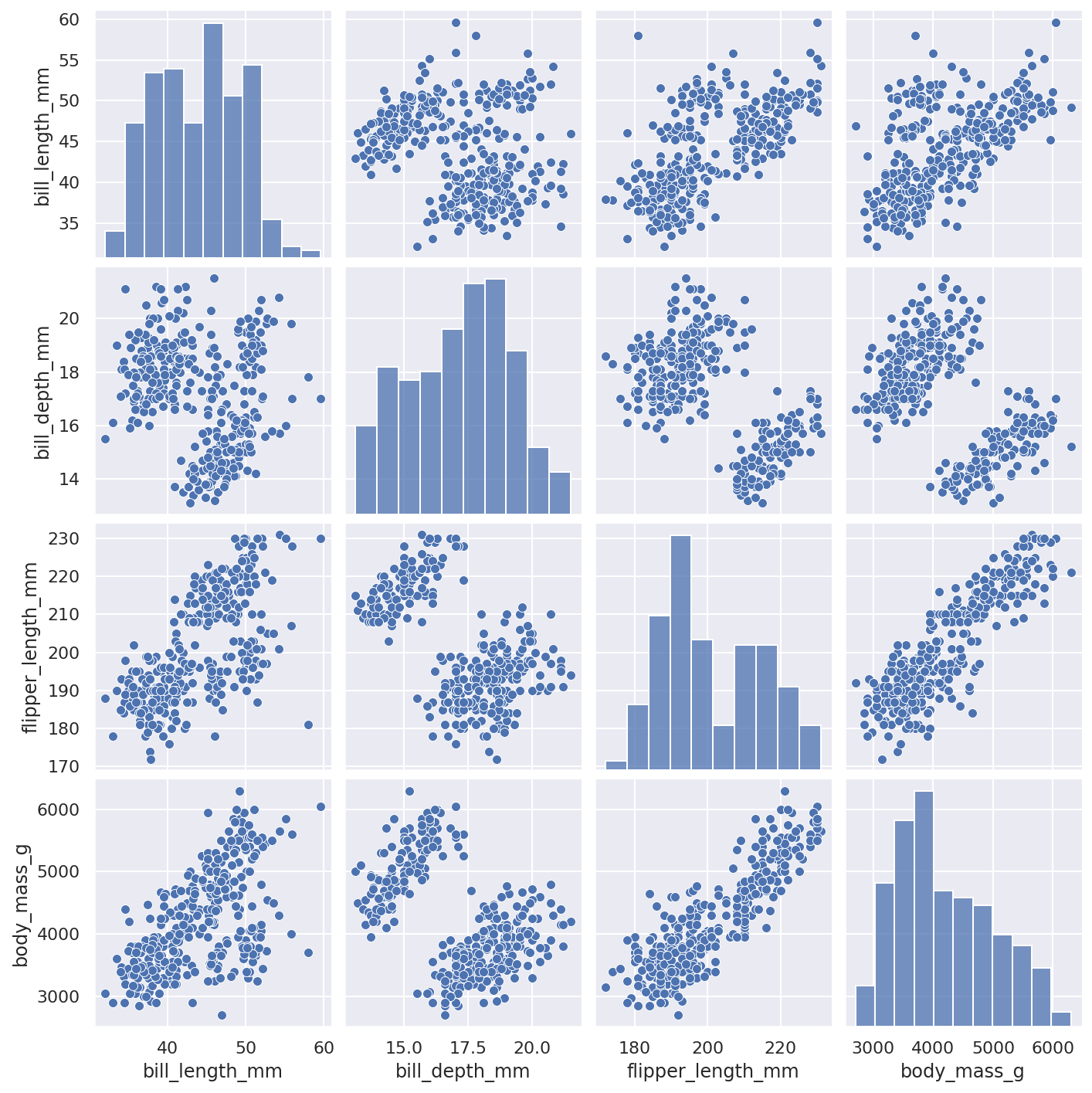#### Adding a “hue” to pairplots

Another really neat feature of Seaborn is the ability to be able to colour code categorical data using the “hue” argument. All you need to do is enter the `hue` argument and tell it the name of the column in the dataframe that contains the categorical variable through which you want to separate the data. It then gives you colour coded plots that tell you even more.

``````sns.pairplot(df, hue='species')
``````
``````<seaborn.axisgrid.PairGrid at 0x7fc23acf24f0>
``````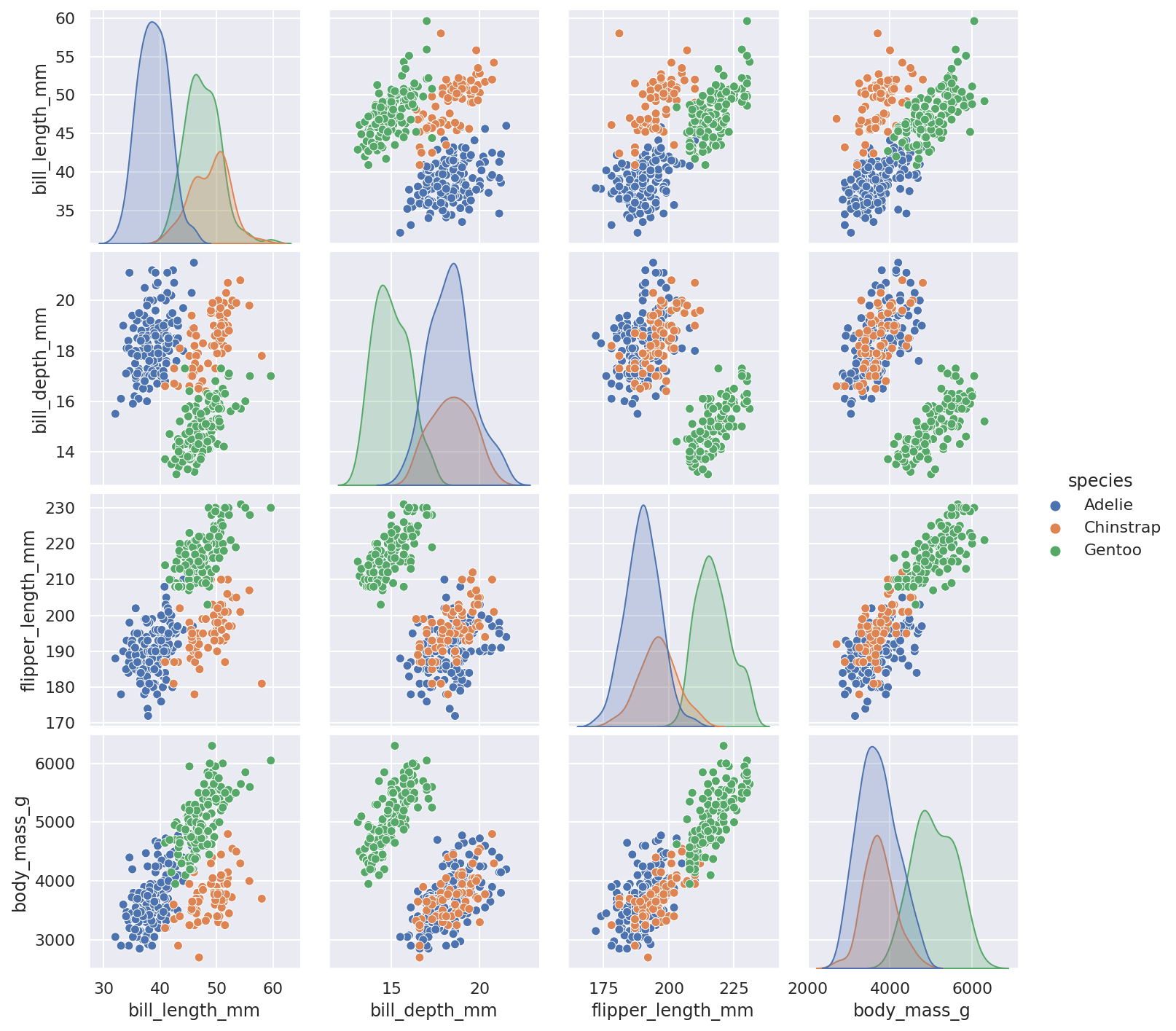### Histograms

There are quite a few ways to analyse the statistical distribution of a single column or variable. The histogram is one of the most commonly used (and appears on the diagonal positions of the pairplot above). These are dead easy to create using the new `displot()` function. Just pass in the dataframe and define the column to plot on the x axis.

``````sns.displot(df, x=df['bill_length_mm'])
``````
``````<seaborn.axisgrid.FacetGrid at 0x7fc238038ca0>
``````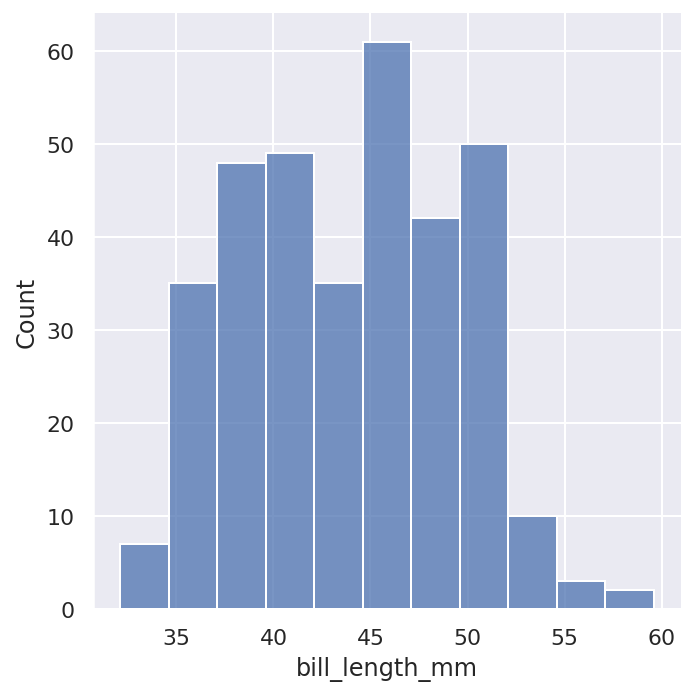As with Pandas histograms, you can specify the number of bins to split the data into using the `bins` argument. This can often be useful if you want a bit more granularity or resolution.

``````sns.displot(df, x=df['bill_length_mm'], bins=20)
``````
``````<seaborn.axisgrid.FacetGrid at 0x7fc23ab65d90>
``````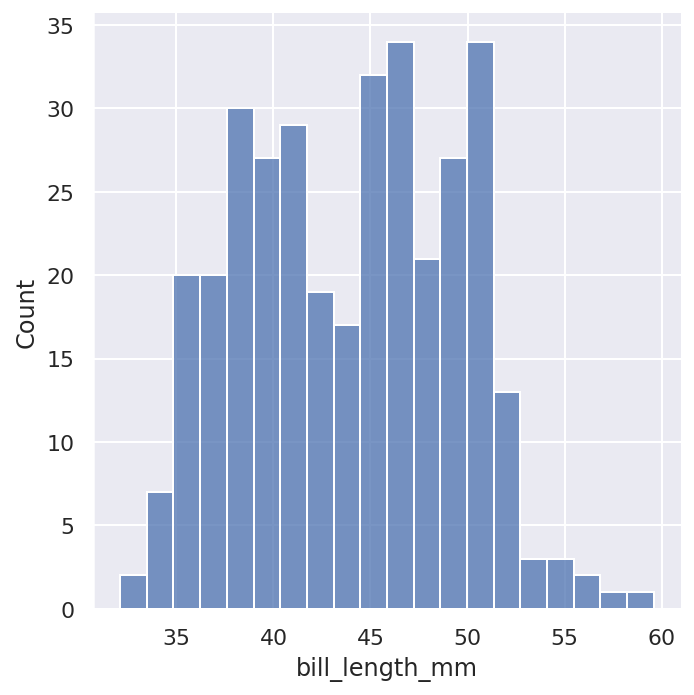By passing the `kde=True` argument you can overlay the standard histogram with a Kernel Density Estimate or KDE plot. The KDE plot basically smooths out the observations, giving you a slightly different view.

``````sns.displot(df, x=df['bill_length_mm'], kde=True)
``````
``````<seaborn.axisgrid.FacetGrid at 0x7fc23aa8eb50>
``````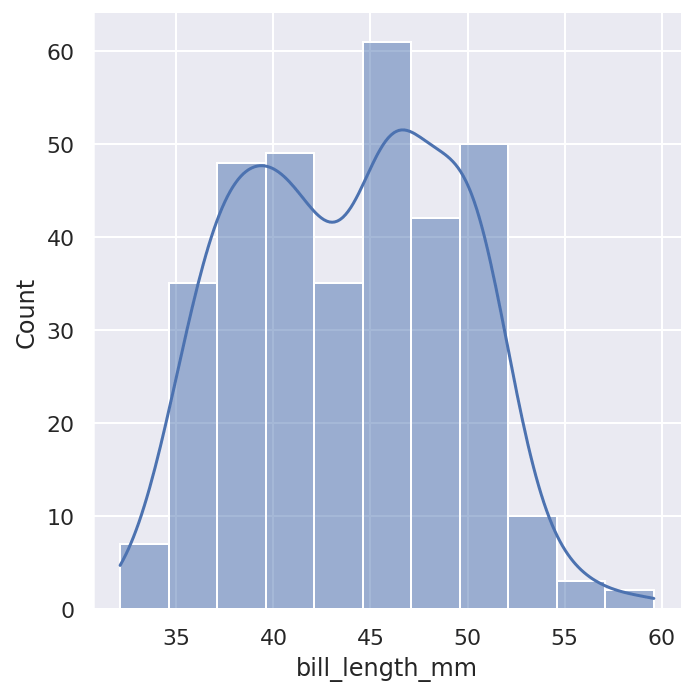To understand how each feature of the penguins differs across species you can use the `hue` argument. This is set to the categorical feature you want to plot (i.e. `species`) and will then plot the species separately in colour coded charts, allowing you to see how the species differ.

``````sns.displot(df, x='flipper_length_mm', hue='species')
``````
``````<seaborn.axisgrid.FacetGrid at 0x7fc23aa65ee0>
``````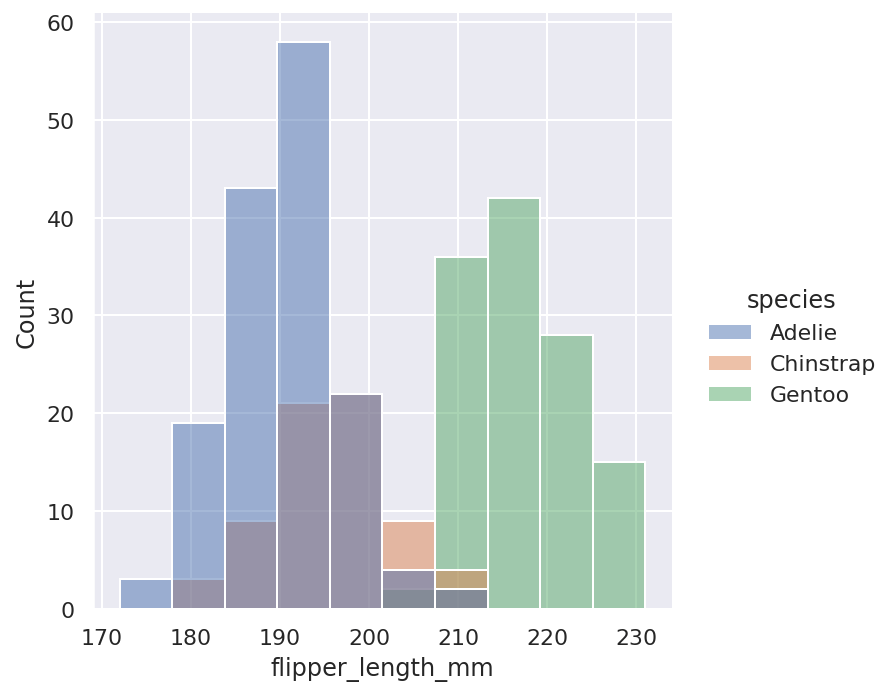As the above style of histogram can make the data harder to read, there’s also an `element='step'` option which turns the regular histogram into a step plot and uses alpha transparency to help you see the underlying overlaps.

``````sns.displot(df, x='bill_depth_mm', hue='species', element='step')
``````
``````<seaborn.axisgrid.FacetGrid at 0x7fc23a9d9e20>
``````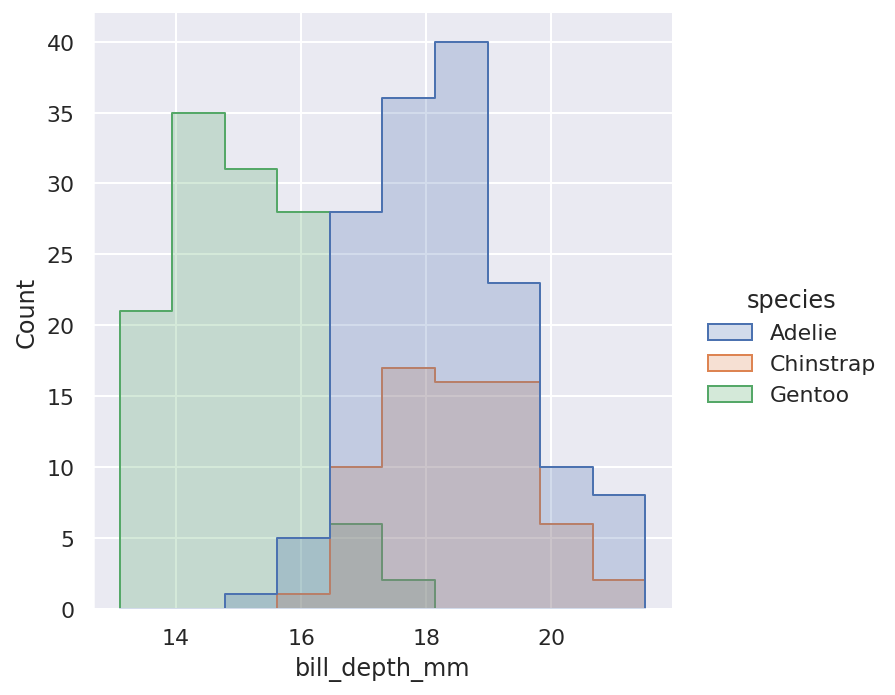If you have a couple of variables, such as the `sex` column, you can view their histogram bars side by side using a combination of the `hue` argument and the `multiple='dodge'` argument.

``````sns.displot(df, x='flipper_length_mm', hue='sex', multiple='dodge')
``````
``````<seaborn.axisgrid.FacetGrid at 0x7fc23a8e7220>
``````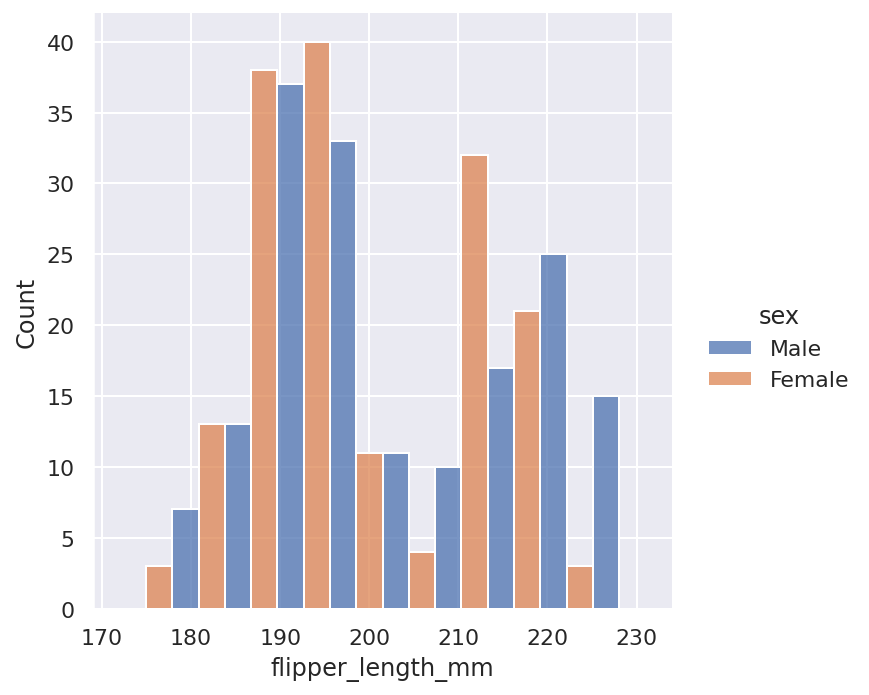Alternatively, if you want to view the two histograms separately side by side, you can use `col` instead of `hue`.

``````sns.displot(df, x='flipper_length_mm', col='sex', multiple='dodge')
``````
``````<seaborn.axisgrid.FacetGrid at 0x7fc23a8dbd60>
``````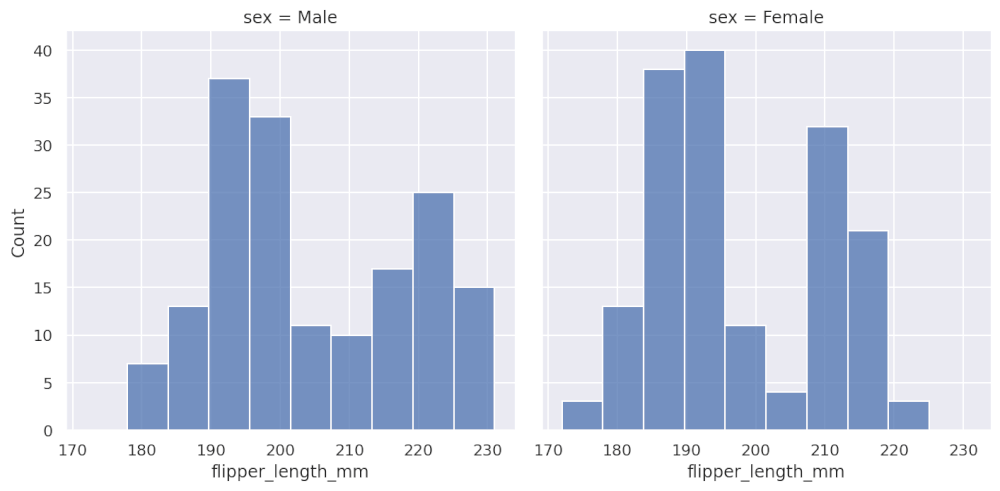### Kernel Density Estimate (KDE) plots

Kernel Density Estimate or KDE plots are a bit like histograms. However, instead of binning data, as histograms do, KDE plots smooth the observations using a “Gaussian kernel” to produce a continuous smoothed estimate.

``````sns.displot(df, x='bill_depth_mm', kind='kde')
``````
``````<seaborn.axisgrid.FacetGrid at 0x7fc23a7628e0>
``````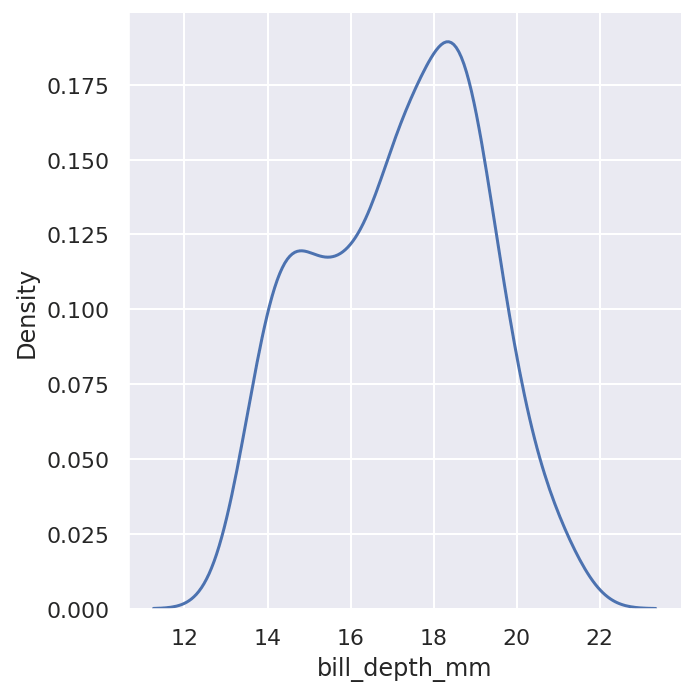To see more of the underlying noise in the data you can reduce the smoothing using the `bw_adjust` argument. Increasing this value does the opposite and gives you an even smoother plot.

``````sns.displot(df, x='bill_depth_mm', kind='kde', bw_adjust=0.5)
``````
``````<seaborn.axisgrid.FacetGrid at 0x7fc23a70bac0>
``````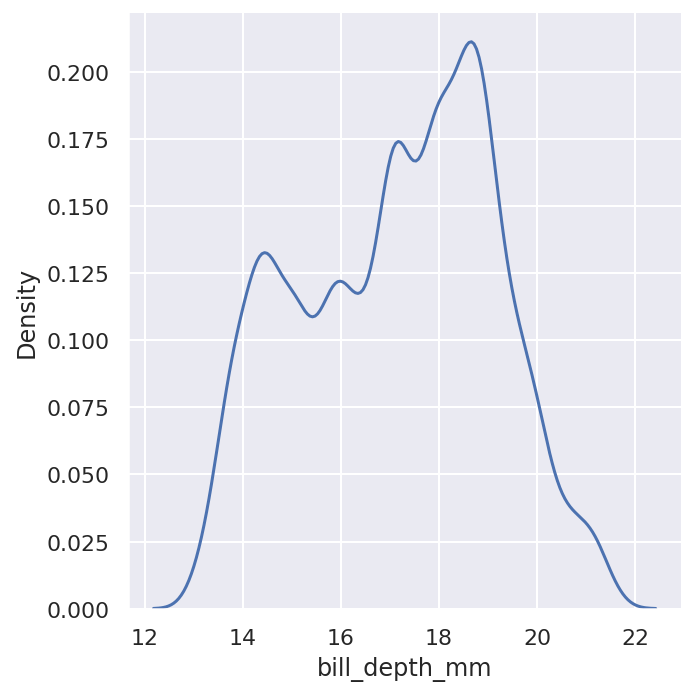As with histograms, you can also use the `hue` argument on `displot()` functions using the KDE plot. These are often a bit easier to read, I think.

``````sns.displot(df, x='bill_depth_mm', kind='kde', hue='species')
``````
``````<seaborn.axisgrid.FacetGrid at 0x7fc23a762bb0>
``````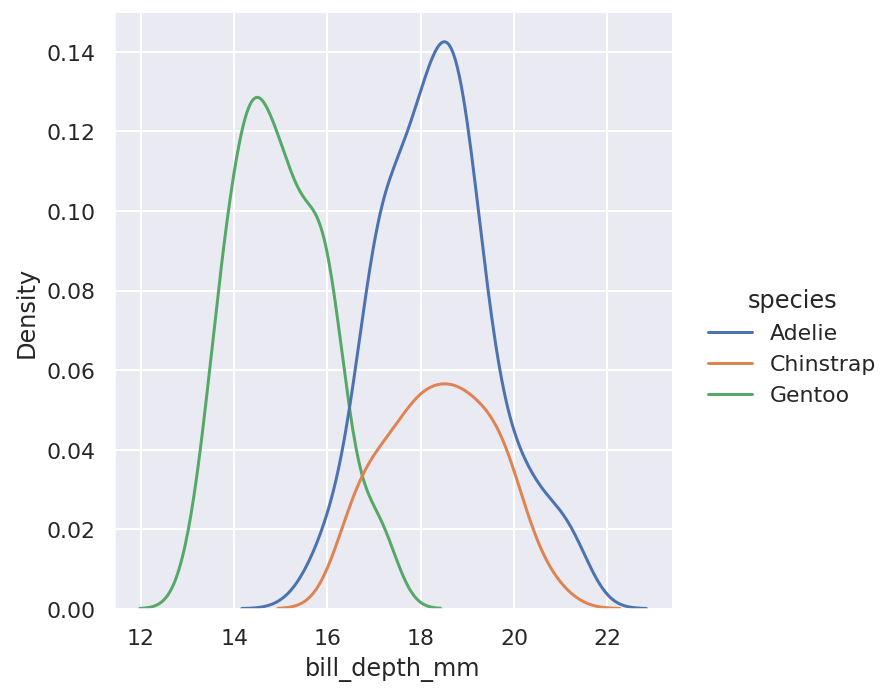### Joint plots

Seaborn also allows you to use several plots at once, allowing the examination of distributions and relationships simultaneously. Here’s a `jointplot()` of the bill length and bill depth for each species, with a KDE plot on the axes.

``````sns.jointplot(data=df, x="bill_length_mm", y="bill_depth_mm", hue="species", kind="kde")
``````
``````<seaborn.axisgrid.JointGrid at 0x7fc23a746b80>
``````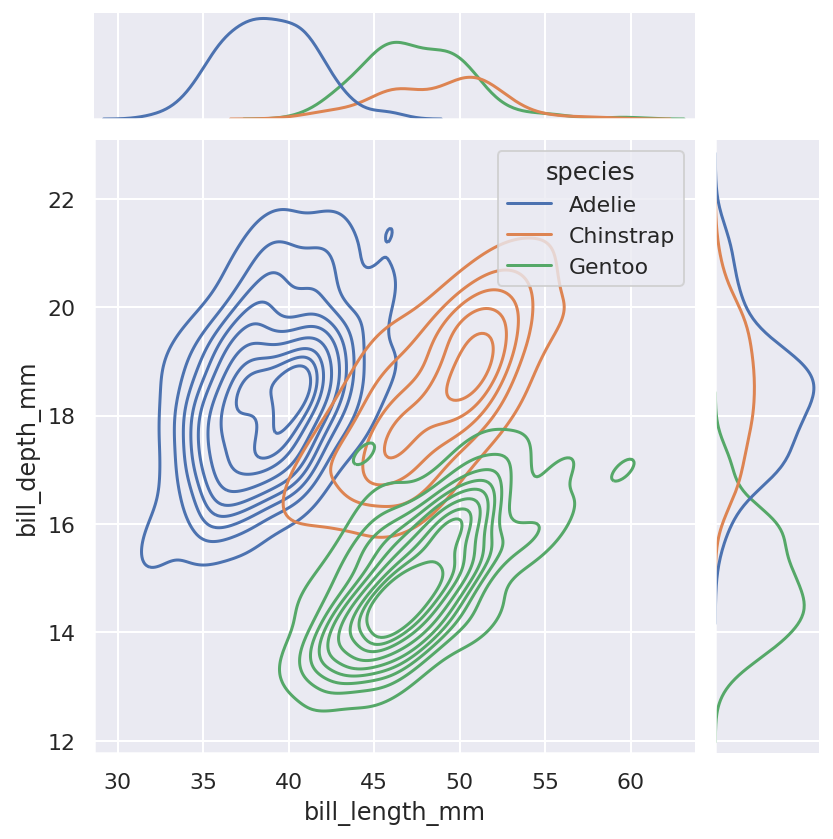### Boxplots

Boxplots are also useful for showing the distribution of data in a quantitative manner, and especially for showing outliers, as seen here on the Gentoo penguin data.

``````sns.boxplot(x=df["species"], y=df["bill_length_mm"])
``````
``````<matplotlib.axes._subplots.AxesSubplot at 0x7fc23a71c310>
``````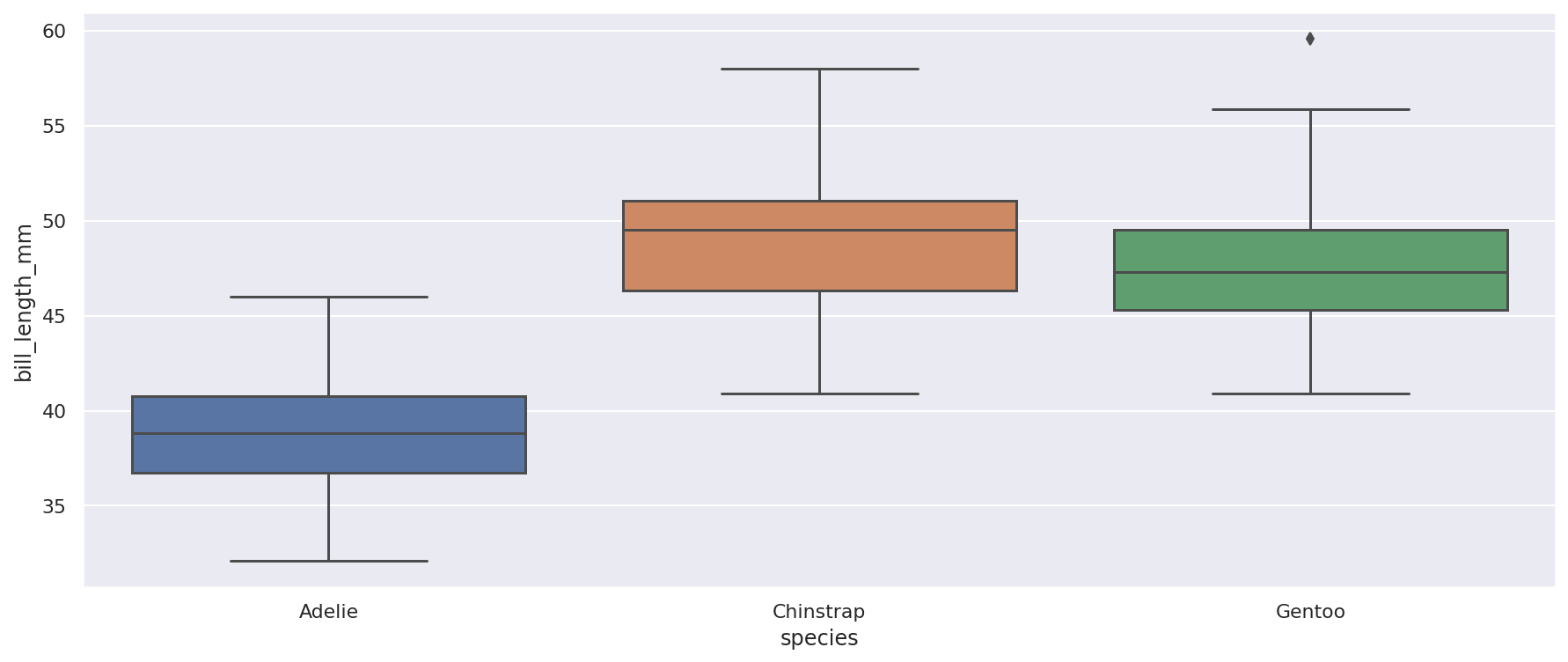### Violinplots

Violinplots are similar to box plots in many ways, and depict the distribution of quantitative data, but the edges incorporate a Kernel Density Estimate showing the spread of data in ways that the boxplot cannot.

``````sns.violinplot(x=df["species"], y=df["bill_length_mm"])
``````
``````<matplotlib.axes._subplots.AxesSubplot at 0x7fc23a5238e0>
``````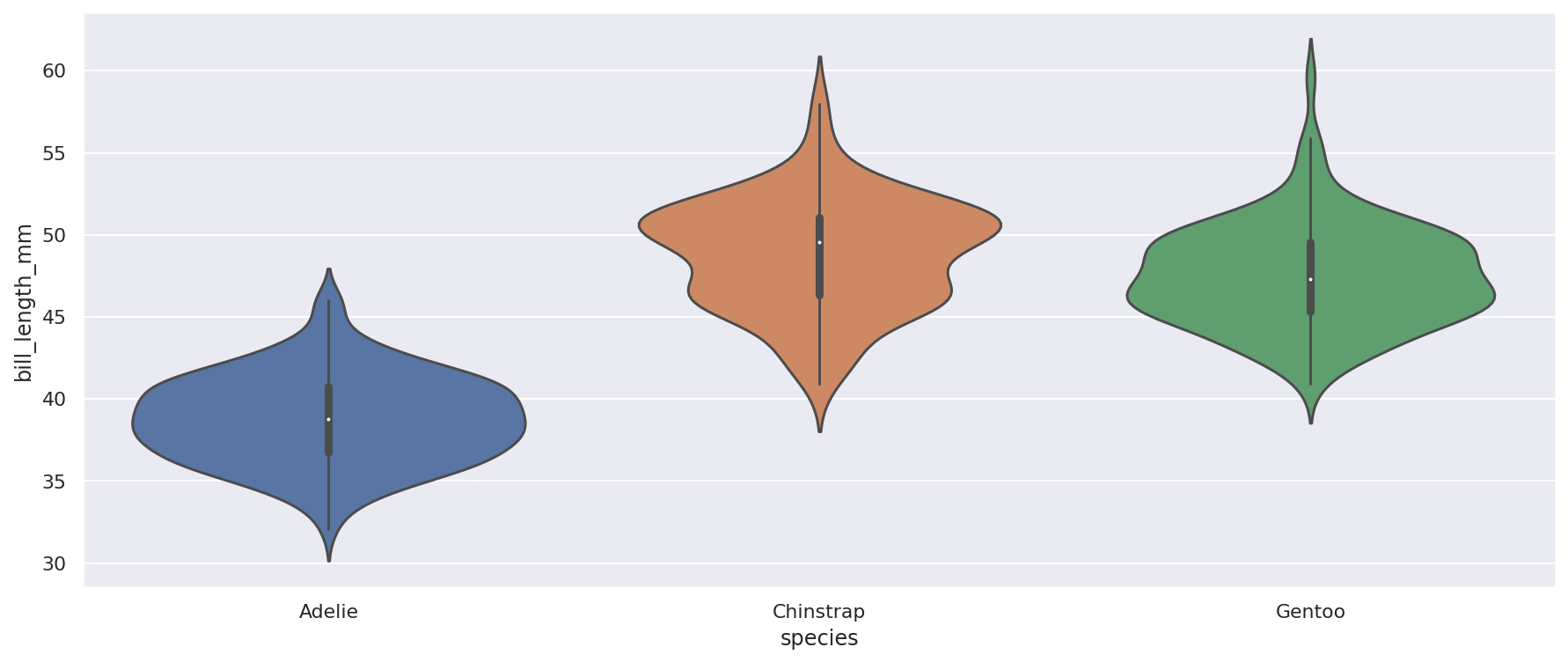Matt Clarke, Sunday, March 07, 2021

Matt Clarke Matt is an Ecommerce and Marketing Director who uses data science to help in his work. Matt has a Master's degree in Internet Retailing (plus two other Master's degrees in different fields) and specialises in the technical side of ecommerce and marketing.